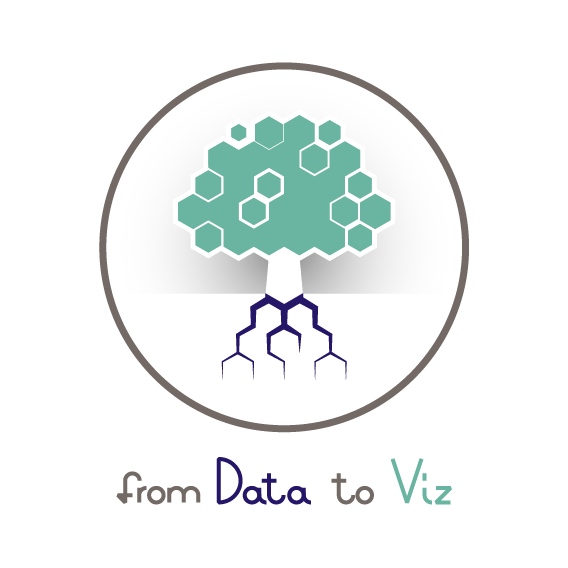# Data Visualization and R

• Data Visualization and R, part 1, Outline
• Files
• Data Visualization and R, part 2, Introduction
• Data Visualization and R, part 3, History

## Data Visualization and R

• Data Visualization and R, part 1, Outline
• Files
• Data Visualization and R, part 2, Introduction
• Data Visualization and R, part 3, History
• Data Visualization and R, part 4, lattice and the small multiple
• Data Visualization and R, part 1, Outline
• Files
• Data Visualization and R, part 2, Introduction
• Data Visualization and R, part 3, History
• Data Visualization and R, part 4, lattice and the small multiple
• Data Visualization and R, part 1, Outline
• Files
• Data Visualization and R, part 2, Introduction
• Data Visualization and R, part 3, History
• Data Visualization and R, part 4, lattice and the small multiple

## Data Visualization and R

• Data Visualization and R, part 1, Outline
• Files
• Data Visualization and R, part 2, Introduction
• Data Visualization and R, part 3, History

### ​from Data to Viz​